# Search by Topic

#### Resources tagged with Place value similar to AB Search:

Filter by: Content type:
Age range:
Challenge level:

### There are 56 results

Broad Topics > Numbers and the Number System > Place value### Just Repeat

##### Age 11 to 14 Challenge Level:

Think of any three-digit number. Repeat the digits. The 6-digit number that you end up with is divisible by 91. Is this a coincidence?### X Marks the Spot

##### Age 11 to 14 Challenge Level:

When the number x 1 x x x is multiplied by 417 this gives the answer 9 x x x 0 5 7. Find the missing digits, each of which is represented by an "x" .### Digit Sum

##### Age 11 to 14 Challenge Level:

What is the sum of all the digits in all the integers from one to one million?### What an Odd Fact(or)

##### Age 11 to 14 Challenge Level:

Can you show that 1^99 + 2^99 + 3^99 + 4^99 + 5^99 is divisible by 5?### Skeleton

##### Age 11 to 14 Challenge Level:

Amazing as it may seem the three fives remaining in the following `skeleton' are sufficient to reconstruct the entire long division sum.### Basically

##### Age 11 to 14 Challenge Level:

The number 3723(in base 10) is written as 123 in another base. What is that base?### Phew I'm Factored

##### Age 14 to 16 Challenge Level:

Explore the factors of the numbers which are written as 10101 in different number bases. Prove that the numbers 10201, 11011 and 10101 are composite in any base.### Arrange the Digits

##### Age 11 to 14 Challenge Level:

Can you arrange the digits 1,2,3,4,5,6,7,8,9 into three 3-digit numbers such that their total is close to 1500?### Even Up

##### Age 11 to 14 Challenge Level:

Consider all of the five digit numbers which we can form using only the digits 2, 4, 6 and 8. If these numbers are arranged in ascending order, what is the 512th number?### Double Digit

##### Age 11 to 14 Challenge Level:

Choose two digits and arrange them to make two double-digit numbers. Now add your double-digit numbers. Now add your single digit numbers. Divide your double-digit answer by your single-digit answer. . . .### Six Times Five

##### Age 11 to 14 Challenge Level:

How many six digit numbers are there which DO NOT contain a 5?### Method in Multiplying Madness?

##### Age 7 to 14 Challenge Level:

Watch our videos of multiplication methods that you may not have met before. Can you make sense of them?### Subtraction Surprise

##### Age 7 to 14 Challenge Level:

Try out some calculations. Are you surprised by the results?### Legs Eleven

##### Age 11 to 14 Challenge Level:

Take any four digit number. Move the first digit to the end and move the rest along. Now add your two numbers. Did you get a multiple of 11?### Three Times Seven

##### Age 11 to 14 Challenge Level:

A three digit number abc is always divisible by 7 when 2a+3b+c is divisible by 7. Why?##### Age 11 to 14 Challenge Level:

Powers of numbers behave in surprising ways. Take a look at some of these and try to explain why they are true.### Lesser Digits

##### Age 11 to 14 Challenge Level:

How many positive integers less than or equal to 4000 can be written down without using the digits 7, 8 or 9?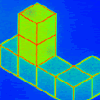### Pupils' Recording or Pupils Recording

##### Age 5 to 14

This article, written for teachers, looks at the different kinds of recordings encountered in Primary Mathematics lessons and the importance of not jumping to conclusions!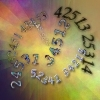### Permute It

##### Age 11 to 14 Challenge Level:

Take the numbers 1, 2, 3, 4 and 5 and imagine them written down in every possible order to give 5 digit numbers. Find the sum of the resulting numbers.### Multiplication Magic

##### Age 14 to 16 Challenge Level:

Given any 3 digit number you can use the given digits and name another number which is divisible by 37 (e.g. given 628 you say 628371 is divisible by 37 because you know that 6+3 = 2+7 = 8+1 = 9). . . .### Really Mr. Bond

##### Age 14 to 16 Challenge Level:

115^2 = (110 x 120) + 25, that is 13225 895^2 = (890 x 900) + 25, that is 801025 Can you explain what is happening and generalise?### What a Joke

##### Age 14 to 16 Challenge Level:

Each letter represents a different positive digit AHHAAH / JOKE = HA What are the values of each of the letters?### Repeaters

##### Age 11 to 14 Challenge Level:

Choose any 3 digits and make a 6 digit number by repeating the 3 digits in the same order (e.g. 594594). Explain why whatever digits you choose the number will always be divisible by 7, 11 and 13.### Not a Polite Question

##### Age 11 to 14 Challenge Level:

When asked how old she was, the teacher replied: My age in years is not prime but odd and when reversed and added to my age you have a perfect square...### Big Powers

##### Age 11 to 16 Challenge Level:

Three people chose this as a favourite problem. It is the sort of problem that needs thinking time - but once the connection is made it gives access to many similar ideas.### Learn about Number Bases

##### Age 11 to 18

We are used to writing numbers in base ten, using 0, 1, 2, 3, 4, 5, 6, 7, 8, and 9. Eg. 75 means 7 tens and five units. This article explains how numbers can be written in any number base.### A Story about Absolutely Nothing

##### Age 7 to 18

This article for the young and old talks about the origins of our number system and the important role zero has to play in it.### Novemberish

##### Age 14 to 16 Challenge Level:

a) A four digit number (in base 10) aabb is a perfect square. Discuss ways of systematically finding this number. (b) Prove that 11^{10}-1 is divisible by 100.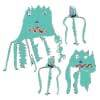### Back to the Planet of Vuvv

##### Age 11 to 14 Challenge Level:

There are two forms of counting on Vuvv - Zios count in base 3 and Zepts count in base 7. One day four of these creatures, two Zios and two Zepts, sat on the summit of a hill to count the legs of. . . .### Quick Times

##### Age 11 to 14 Challenge Level:

32 x 38 = 30 x 40 + 2 x 8; 34 x 36 = 30 x 40 + 4 x 6; 56 x 54 = 50 x 60 + 6 x 4; 73 x 77 = 70 x 80 + 3 x 7 Verify and generalise if possible.### Always a Multiple?

##### Age 11 to 14 Challenge Level:

Think of a two digit number, reverse the digits, and add the numbers together. Something special happens...### Seven Up

##### Age 11 to 14 Challenge Level:

The number 27 is special because it is three times the sum of its digits 27 = 3 (2 + 7). Find some two digit numbers that are SEVEN times the sum of their digits (seven-up numbers)?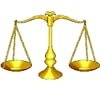### Balance Power

##### Age 11 to 18 Challenge Level:

Using balancing scales what is the least number of weights needed to weigh all integer masses from 1 to 1000? Placing some of the weights in the same pan as the object how many are needed?### Number Rules - OK

##### Age 14 to 16 Challenge Level:

Can you convince me of each of the following: If a square number is multiplied by a square number the product is ALWAYS a square number...### Mini-max

##### Age 11 to 14 Challenge Level:

Consider all two digit numbers (10, 11, . . . ,99). In writing down all these numbers, which digits occur least often, and which occur most often ? What about three digit numbers, four digit numbers. . . .### Enriching Experience

##### Age 14 to 16 Challenge Level:

Find the five distinct digits N, R, I, C and H in the following nomogram### Cayley

##### Age 11 to 14 Challenge Level:

The letters in the following addition sum represent the digits 1 ... 9. If A=3 and D=2, what number is represented by "CAYLEY"?##### Age 11 to 14 Challenge Level:

Replace the letters with numbers to make the addition work out correctly. R E A D + T H I S = P A G E### How Many Miles to Go?

##### Age 11 to 14 Challenge Level:

How many more miles must the car travel before the numbers on the milometer and the trip meter contain the same digits in the same order?### More Mathematical Mysteries

##### Age 11 to 14 Challenge Level:

Write down a three-digit number Change the order of the digits to get a different number Find the difference between the two three digit numbers Follow the rest of the instructions then try. . . .### Tis Unique

##### Age 11 to 14 Challenge Level:

This addition sum uses all ten digits 0, 1, 2...9 exactly once. Find the sum and show that the one you give is the only possibility.### Latin Numbers

##### Age 14 to 16 Challenge Level:

Can you create a Latin Square from multiples of a six digit number?### Cycle It

##### Age 11 to 14 Challenge Level:

Carry out cyclic permutations of nine digit numbers containing the digits from 1 to 9 (until you get back to the first number). Prove that whatever number you choose, they will add to the same total.### Football Sum

##### Age 11 to 14 Challenge Level:

Find the values of the nine letters in the sum: FOOT + BALL = GAME### Eleven

##### Age 11 to 14 Challenge Level:

Replace each letter with a digit to make this addition correct.### DOTS Division

##### Age 14 to 16 Challenge Level:

Take any pair of two digit numbers x=ab and y=cd where, without loss of generality, ab > cd . Form two 4 digit numbers r=abcd and s=cdab and calculate: {r^2 - s^2} /{x^2 - y^2}.### Never Prime

##### Age 14 to 16 Challenge Level:

If a two digit number has its digits reversed and the smaller of the two numbers is subtracted from the larger, prove the difference can never be prime.### Nice or Nasty for Two

##### Age 7 to 14 Challenge Level:

Some Games That May Be Nice or Nasty for an adult and child. Use your knowledge of place value to beat your opponent.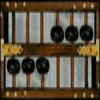### Adding with the Abacus

##### Age 5 to 16

Nowadays the calculator is very familiar to many of us. What did people do to save time working out more difficult problems before the calculator existed?### 2-digit Square

##### Age 14 to 16 Challenge Level:

A 2-Digit number is squared. When this 2-digit number is reversed and squared, the difference between the squares is also a square. What is the 2-digit number?